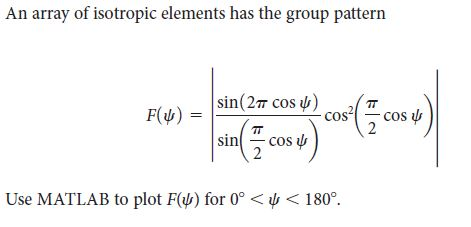# An array of isotropic elements has the group patternsin(27 cos u)cos-cos uF(4)sin- cos uUse MATLAB to plot F() for 0° < y < 180°.

Question
6 views

MATLAB code with output- Refer Book Electromagnetic theory by Mathew. N.O. Sadiku-7th edition - Chapter 13-Problem 13.39help_outlineImage TranscriptioncloseAn array of isotropic elements has the group pattern sin(27 cos u) cos -cos u F(4) sin - cos u Use MATLAB to plot F() for 0° < y < 180°. fullscreen
check_circle

Step 1

In this case, the group pattern of the array of isotropic elements is given as

Step 2

The corresponding MATLAB code explaining all the necessary steps to plot the group pattern is given below:

psi=0:0.0001:180; %creates a vector psi with the angular values between 0 and 180 with spacing of 0.0001

%the group pattern comprises of 3 different terms

%the three terms are constructed in g, m and n

g=sin(2*pi*cos(psi));

m=sin((pi/2)*cos(psi));

n=cos((pi/2)*cos(psi)).^2;

F_psi=abs((g/m)*n); %abs stands for absolute value of magnitude in MATLAB

plot(psi,F_psi) %this plots the graph of F_psi v/s psi.

title('Plot of Group Pattern')

xlabel('Angle in degree')

ylabel('Amplitude')

...

### Want to see the full answer?

See Solution

#### Want to see this answer and more?

Solutions are written by subject experts who are available 24/7. Questions are typically answered within 1 hour.*

See Solution
*Response times may vary by subject and question.
Tagged in

### Electrical Engineering